List

This post aims to summarize the main concepts that are involved in a symbol timing synchronization scheme by exploring a MATLAB example. For the reader interested in going deeper into the topic, I definitely recommend Michael Rice’s digital communications book , the very book that is taken as reference in MATLAB’s symbol timing synchronization implementation. After understanding the concepts presented there, I recommend exploring the MATLAB simulation that I developed and published on Github:

Background

What is symbol timing synchronization?

This should obviously be the first thing to be understood. Since there are many flavors of synchronization, the precise definition of each synchronization can become confusing. For example, there is clock synchronization, frame synchronization, carrier synchronization, all of which are different concepts with respect to symbol timing synchronization.

Symbol timing synchronization has a very unique purpose: to find the optimal instants when downsampling a sequence of receive samples into receive symbols, such that the “best” symbols are passed to the symbol detector. This will become crystal clear once we explore the forthcoming example.

Components of a Symbol Timing Recovery Loop

To understand the components of a timing recovery loop, it is useful to revisit what a basic feedback control loop is. In essence, a control loop has three main elements: an error detector, a filter and the “plant” (or “process”). It is informative to recall the role of each element, and then understand what are those elements in the context of a symbol timing synchronization loop.

The goal of a feedback control system is to control the response to a certain input signal. For example, when a power switch is turned on (say from 0 to 12V), such system could be used to control the pace in which the output voltage switches from 0 to 12V, for example, by guaranteeing that it settles at 12V steadily within a given time specification. The error detector compares the input and the output of the loop. The difference (the detected error), is filtered by a block that is known as the “controller”. This controller has several parameters that can be tuned to control the desired output response (for example the just mentioned settling time). Finally, the filter output feeds the “plant”, which should generate a signal that follows the input. As the plant’s outputs gets closer to the input, the error decreases and the system approaches its steady state.

Timing recovery loops are just that, but with their peculiar error detector, filter and plant. Next, a very brief overview of the timing recovery loop elements is given. Do not worry if their essence is not clear yet, it will soon be once we advance into the MATLAB example discussion.

Timing Error Detector

First, note the n-th receive symbol can be modeled by:$y(nT_s) = \sum \limits_{m}x(m)p((n-m)T_s - \tau) + v(nT_s),$

where$T_s$ is the symbol period,$p$ is the channel pulse response (combining both the pulse shaping filter and the receiver-side matched filter),$x(m)$ is the m-th transmit symbol,$v(nT_s)$ is the AWGN and, importantly,$\tau$ is timing offset error within$[0, T_s)$, that is, within a fractional symbol period.

The timing offset error$\tau$ results from the channel propagation delay, which can not be controlled and, therefore, introduces delays that are not simply integer multiples of the symbol period. In reality, the propagation delay is such that$\tau$ is composed by two parcels, one that is an integer multiple of$T_s$ and another that corresponds the fractional multiple of$T_s$. In the context of symbol timing recovery, we are only concerned with the fractional error. The integer error is left to be corrected by a frame synchronization scheme.

It is only because of the timing error$\tau$ that the parcels for$n \neq m$ in the summation of the above expression for$y(nT_s)$ are non-zero. Therefore, due to$\tau$, intersymbol interference affects the symbol. This will also be clarified within the MATLAB example presented soon.

The timing error detector has the purpose of estimating this timing error$\tau$, so that the receiver can adjust its timing and avoid the intersymbol interference.

Loop Filter

The essence of the loop filter is that it is designed to control how fast the timing error can be corrected, what types of timing error can be corrected (for example linearly varying) and how large of a timing error can be corrected. In general, it is a second-order system and often the Proportional-plus-Integral controller (PI controller), which is commonly used in feedback systems. In this post, we choose not to give deep details about the loop filter. Refer to  (specially in Appendix C) for a careful treatment about this element.

Interpolator Controller and Interpolator

Finally, the two modules that play the role of the plant/process in the context of the symbol timing recovery loop is the interpolator and specially the interpolator controller. The latter dictates the instants along the matched-filter output sequence that should be deemed as the symbols. For an oversampling factor of$L$, there are$L$ samples for each symbol in the output of the MF. The receiver has to pick only one of each$L$ and pass it to the symbol detector. Hence, it can be interpreted that the interpolator controller generates a train of spaced impulses, with impulses solely at the indexes that correspond to the symbols.

The interpolator and its controller won’t be discussed in this tutorial, so, again, the reader is referred to .

In the end, a timing recovery loop generally looks similar to the following diagram: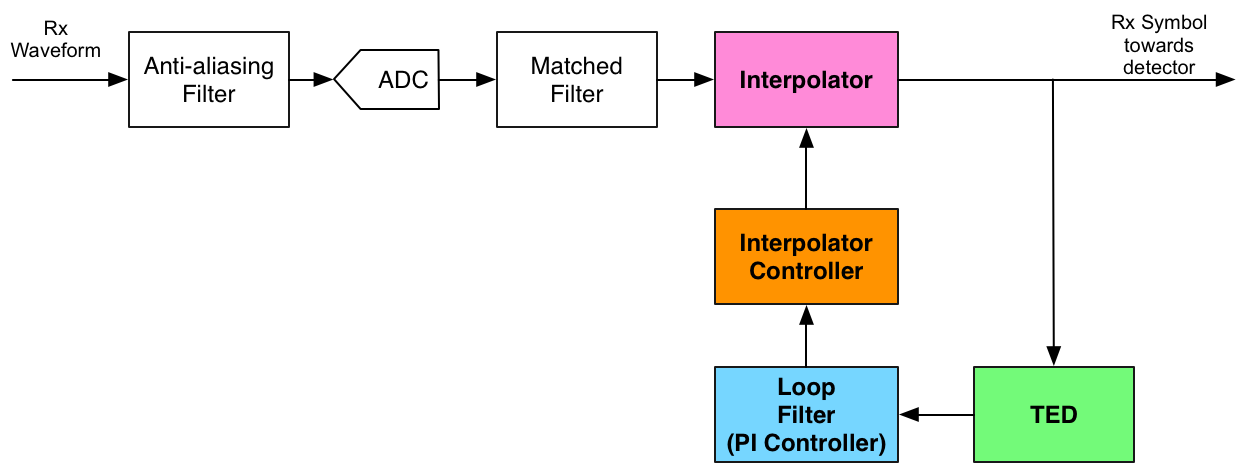Now, I believe we are ready to advance into the actual tutorial. By looking into MATLAB code and corresponding plots, hopefully the main aspects of the timing recovery loop will become clear.

Pulse Shaping Filter Design Considerations

First, design a Square-root Raised Cosine filter for pulse shaping:

L        = 4;         % Oversampling factor
rollOff  = 0.5;        % Pulse shaping roll-off factor
rcDelay  = 10;         % Raised cosine delay in symbols

% Filter:
htx = rcosine(1, L, 'sqrt', rollOff, rcDelay/2);
% Note half of the target delay is used, because when combined
% to the matched filter, the total delay will be achieved.
hrx  = conj(fliplr(htx));

figure
plot(htx)
title('Transmit Filter')
xlabel('Index')
ylabel('Amplitude')

figure
plot(hrx)
title('Rx Filter (Matched Filter)')
xlabel('Index')
ylabel('Amplitude')

p = conv(htx,hrx);

figure
plot(p)
title('Combined Tx-Rx = Raised Cosine')
xlabel('Index')
ylabel('Amplitude')

% And let's highlight the zero-crossings
zeroCrossings = NaN*ones(size(p));
zeroCrossings(1:L:end) = 0;
zeroCrossings((rcDelay)*L + 1) = NaN; % Except for the central index
hold on
plot(zeroCrossings, 'o')
legend('RC Pulse', 'Zero Crossings')
hold off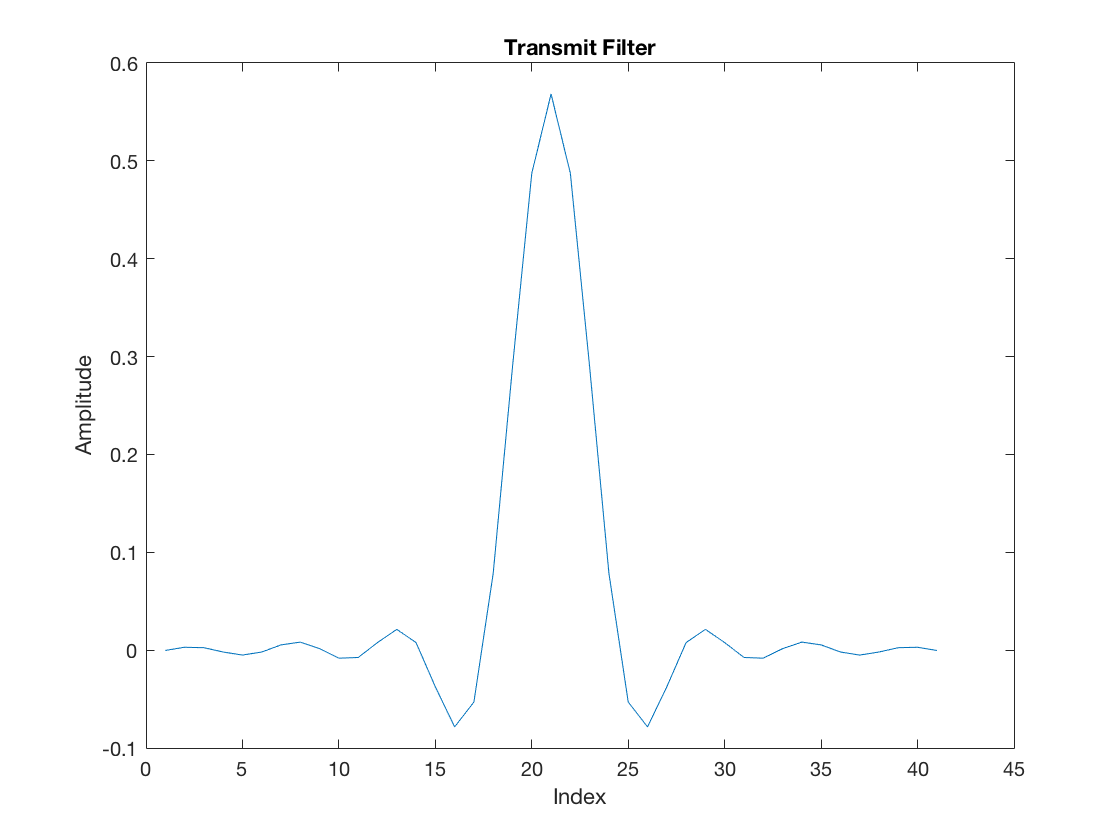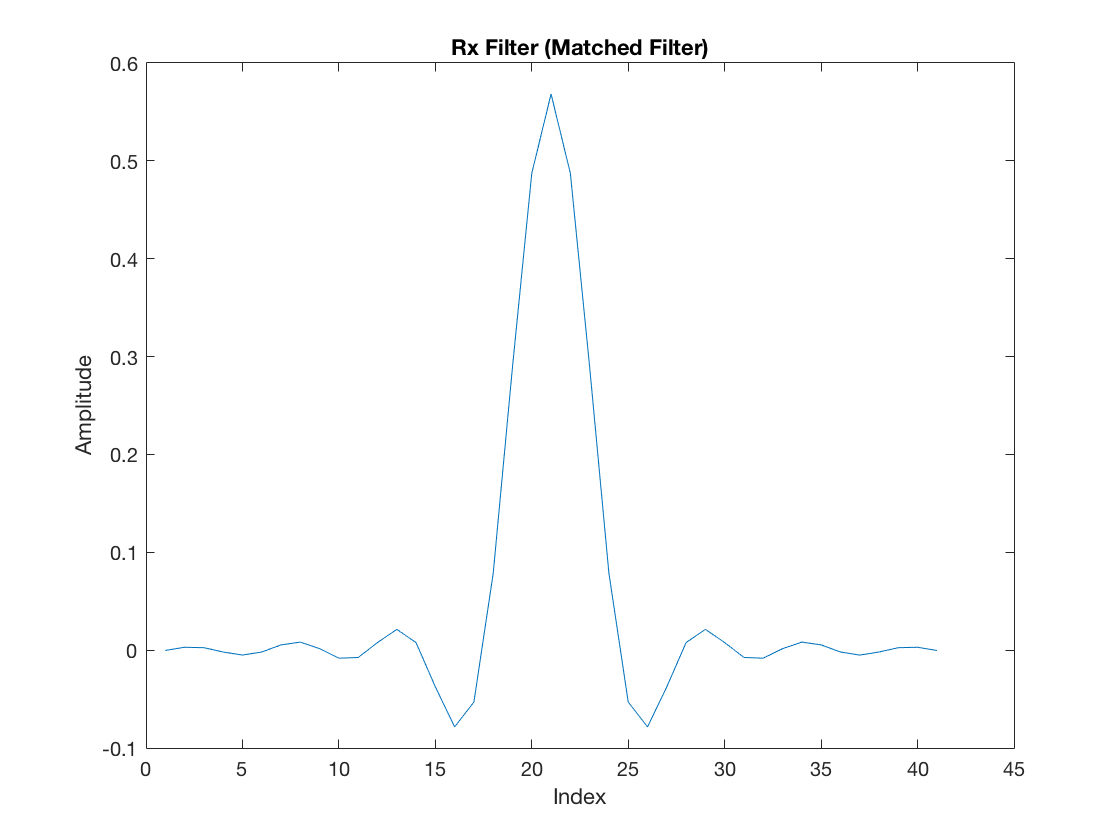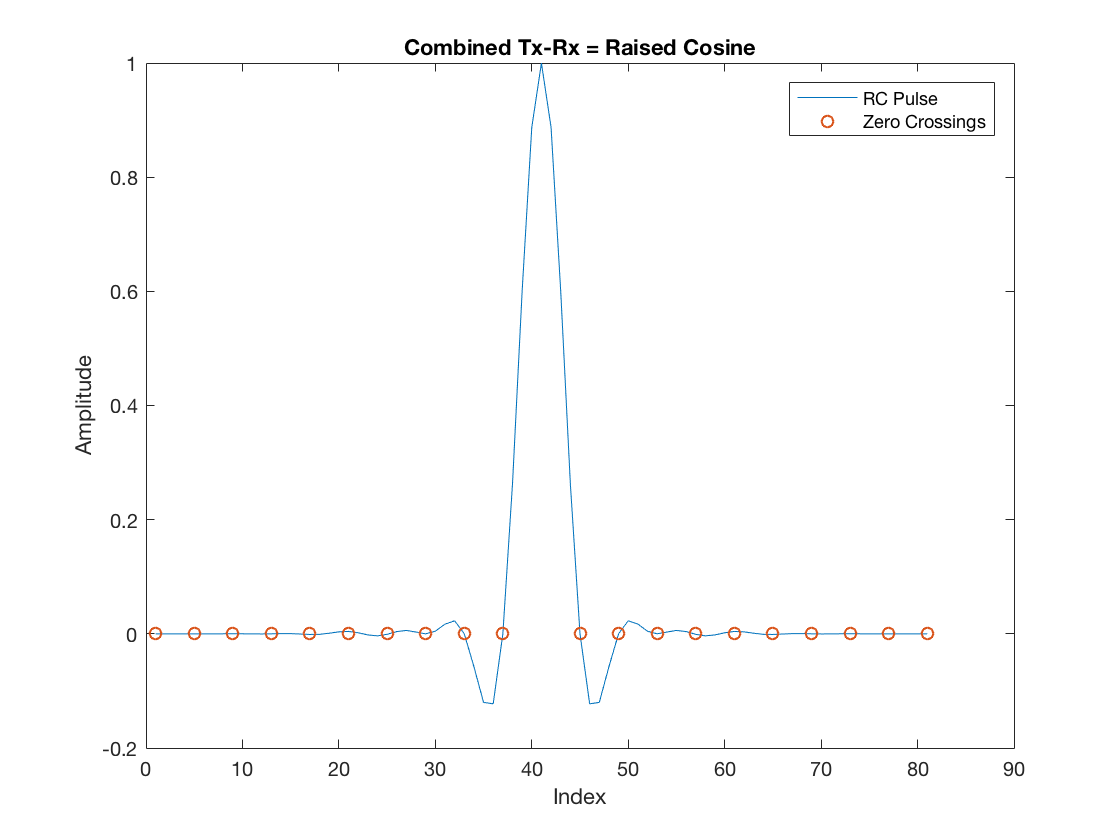The zero-crossings highlighted in the RC pulse are very important in the context of symbol timing synchronization. Once the receiver samples the incoming waveform and performs matched-filtering, the samples that must be “retained” as symbols must be exactly aligned with these zero-crossing instants. This is because by doing so, intersymbol interference is eliminated. That is, a given symbol is multiplied by the RC peak (unitary in this case), and its interference contribution to all other neighbor symbols is exactly the amplitude at the zero-crossings, namely null. In the sequel, this is better illustrated.

Transmitter

In this example, we will observe the transmission of a sequence of 2-PAM symbols. Let’s say we transmit a couple of consecutive PAM symbols over a period that at least is longer than the RC delay:

M             = 2; % Pam Order

% Arbitrary binary data
data          = zeros(1, 2*rcDelay);
data(1:2:end) = M-1;

% PAM-modulated symbols:
txSym         = real(pammod(data, M));

figure
stem(txSym)
title('Symbol Sequence')
xlabel('Symbol Index')
ylabel('Amplitude')And memorize one thing: this sequence was purposely generated with alternating +1, -1, +1, -1 and so forth. First, this will help with visualization. But second and more importantly, this will be very critical soon when we discuss a specific timing error detector scheme. Keep that in mind.

% Upsampling
txUpSequence = upsample(txSym, L);

figure
stem(txUpSequence)
title('Upsampled Sequence')
xlabel('Sample Index')
ylabel('Amplitude')

% Pulse Shaping
txSequence = filter(htx, 1, txUpSequence);

figure
stem(txSequence)
title('Shaped Transmit Sequence')
xlabel('Index')
ylabel('Amplitude')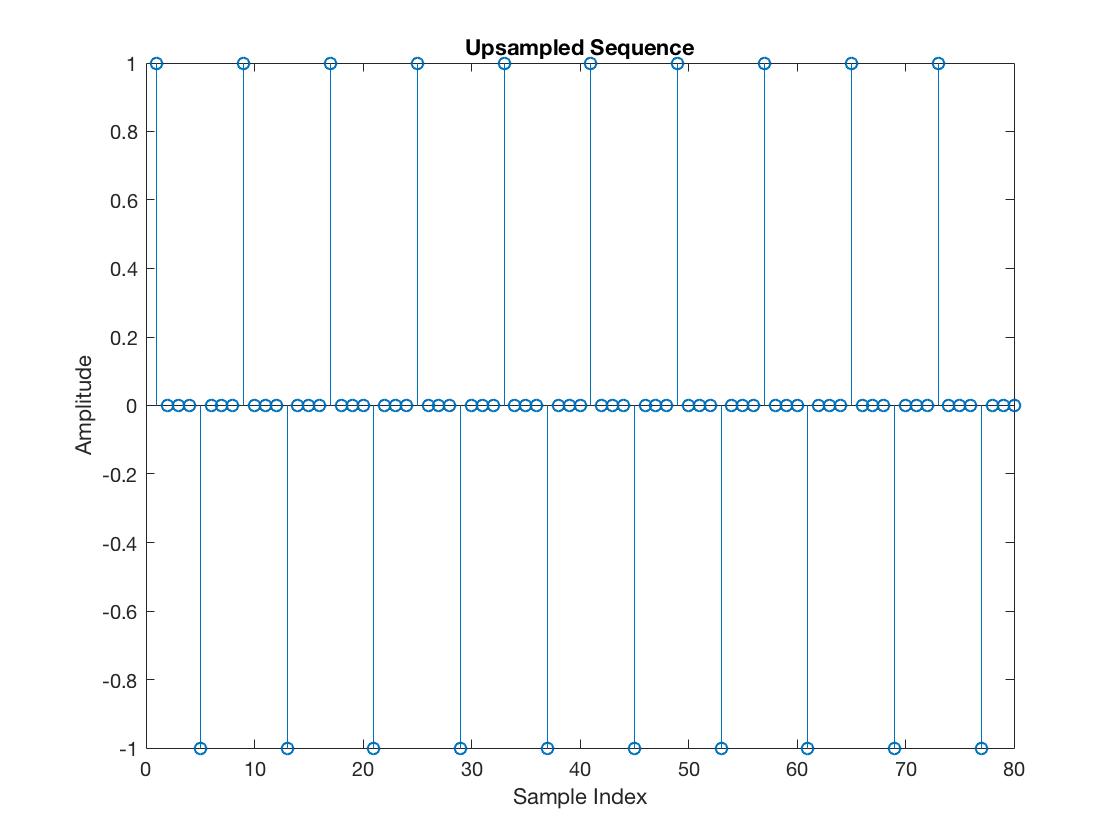Channel

Let’s add a random channel propagation delay in units of sampling intervals (not symbol intervals):

timeOffset = 1; % Delay (in samples) added

% Delayed sequence
rxDelayed = [zeros(1, timeOffset), txSequence(1:end-timeOffset)];


Furthermore, let’s completely ignore AWG noise. This will make the symbol timing synchronization results more prominent and help the explanation.

First, let’s consider a receiver that does not perform symbol timing synchronization. Once the delayed noisy waveform reaches the digital receiver chain, it is first filtered by the matched filter:

mfOutput = filter(hrx, 1, rxDelayed); % Matched filter output

figure
stem(mfOutput)
xlabel('Index')
ylabel('Amplitude')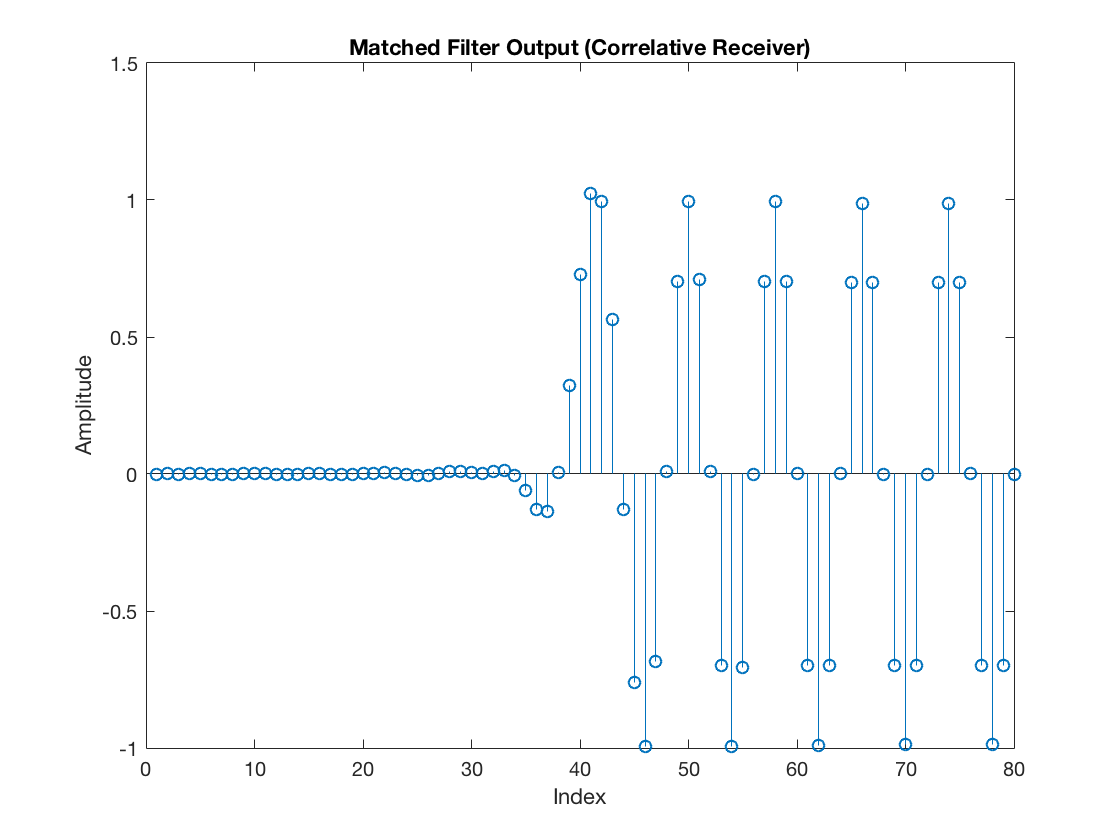Now let’s add an arbitrary timing used by the receiver to select samples from the incoming sequence and pass to the decision module (namely for the downsampler).

rxSym = downsample(mfOutput, L);

% Generate a vector that shows the selected samples
selectedSamples = upsample(rxSym, L);
selectedSamples(selectedSamples == 0) = NaN;

% And just for illustration purposes
figure
stem(mfOutput)
hold on
stem(selectedSamples, '--r', 'LineWidth', 2)
xlabel('Index')
ylabel('Amplitude')
legend('MF Output', 'Downsampled Sequence (Symbols)')
hold off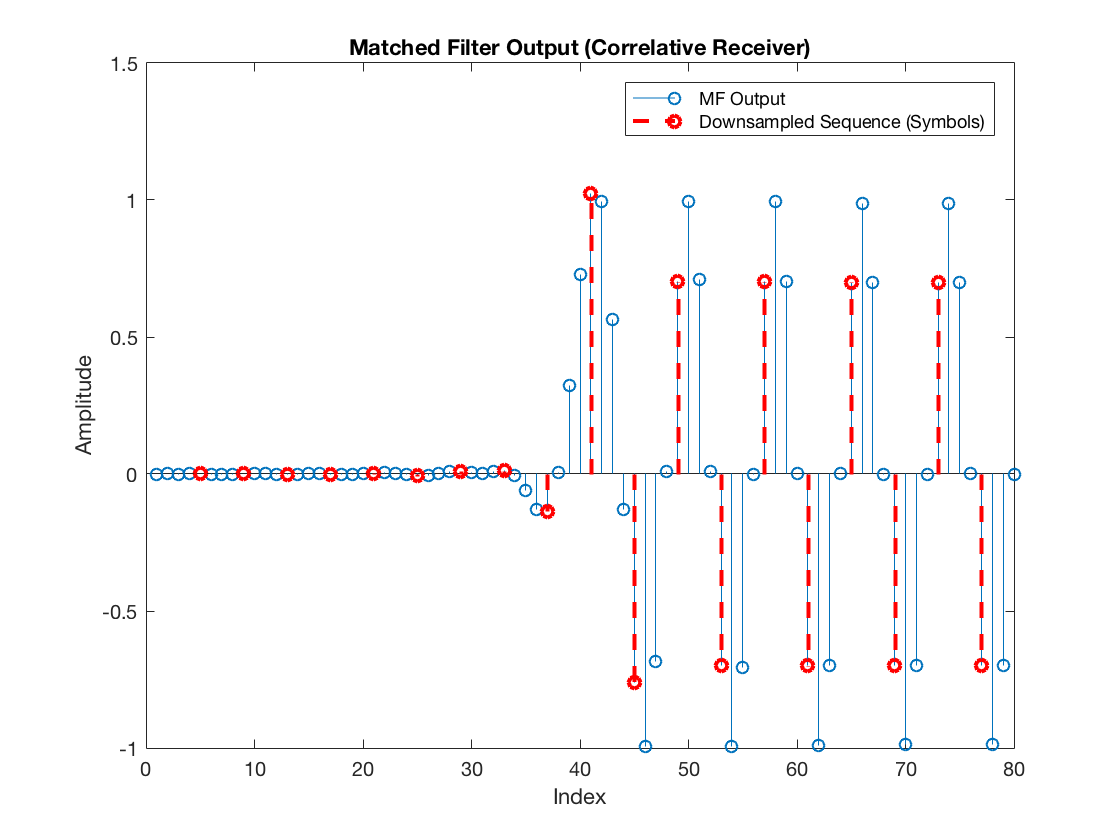And let’s see how these extracted samples (the received symbols) look:

figure
stem(rxSym)
title('Symbol Sequence')
xlabel('Symbol Index')
ylabel('Amplitude')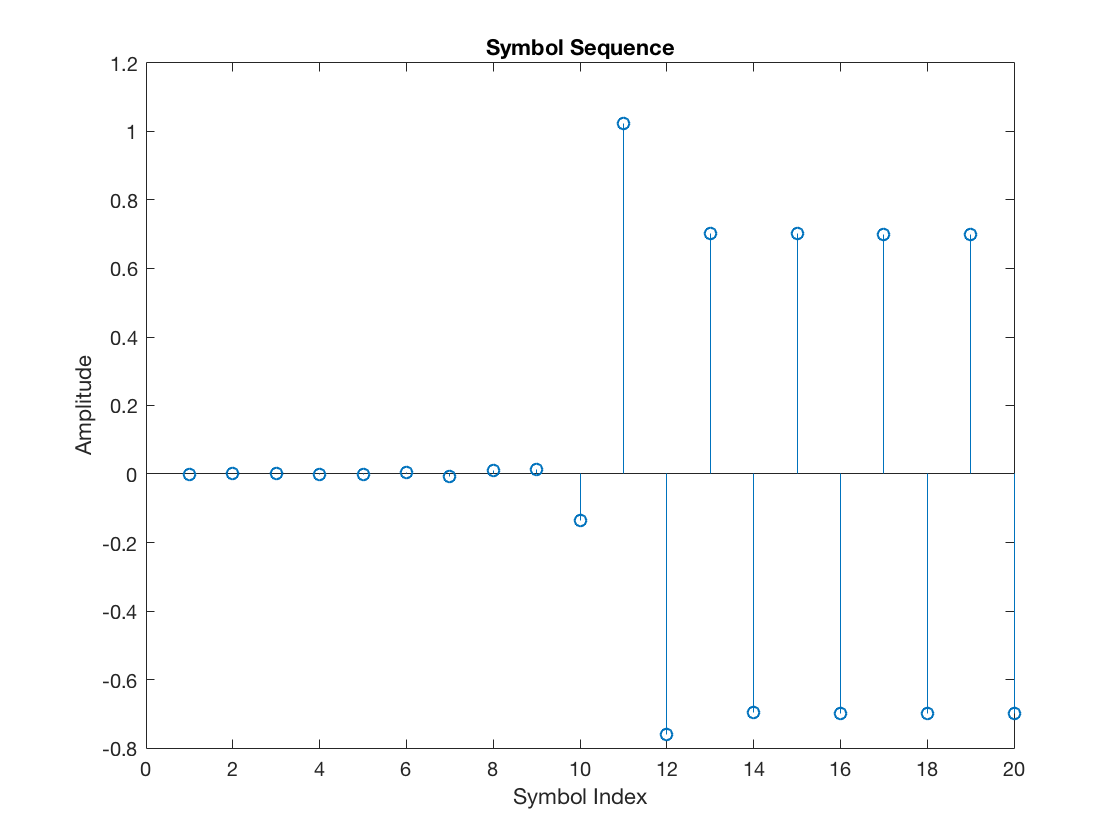Finally, skiping the raised cosine pulse delay, the corresponding scatter plot becomes:

figure
plot(complex(rxSym(rcDelay+1:end)), 'o')
grid on
xlim([-1.5 1.5])
title('Rx Scatterplot')
xlabel('In-phase (I)')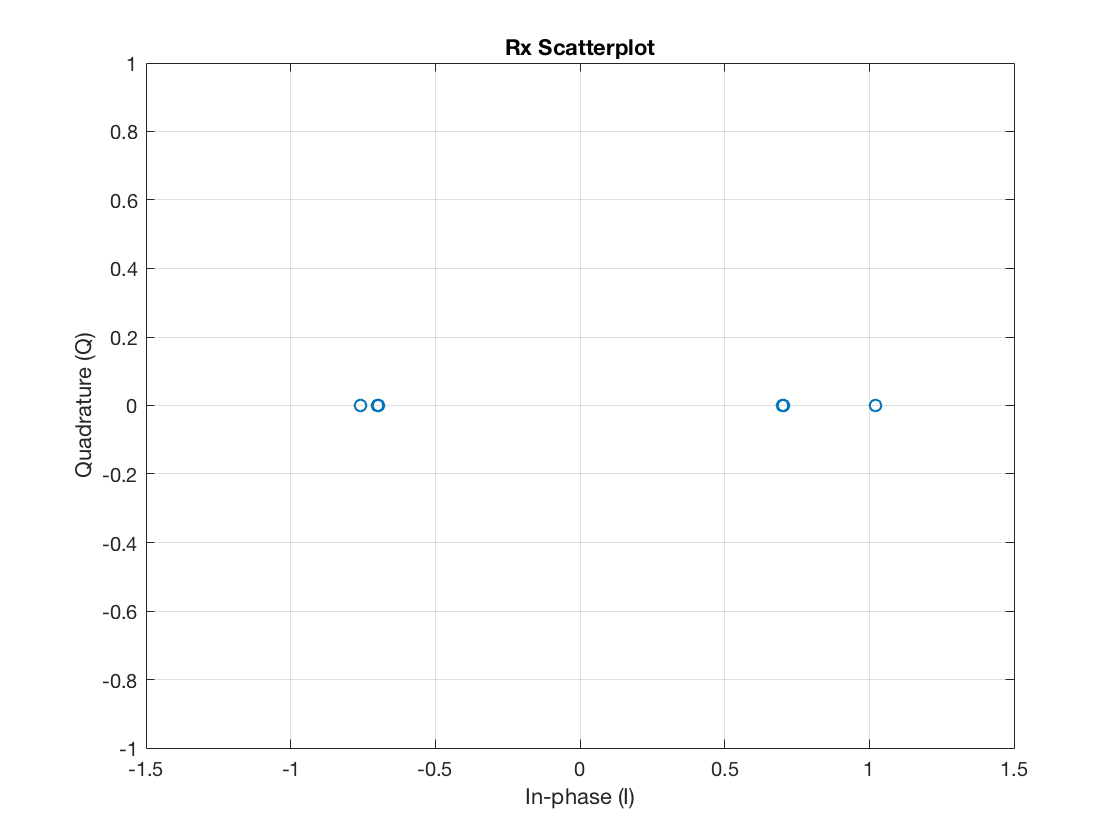Note it does not look good. This is due to the fact that the receiver is not extracting the samples that are aligned with the zero-crossings of the pulse shaping function, so that there is intersymbol interference.

Receiver featured with Symbol Timing Synchronization

Now let’s suppose the receiver knows the symbol timing offset exactly, in terms of sampling periods or, equivalently, fractional symbol intervals. Let’s add the timeOffset variable to the offset argument of the downsample function:

rxSym = downsample(mfOutput, L, timeOffset);


In this case, we can see that the “selected” samples are:

selectedSamples = upsample(rxSym, L);
selectedSamples(selectedSamples == 0) = NaN;

% And just for illustration purposes
figure
stem(mfOutput)
hold on
stem(selectedSamples, '--r', 'LineWidth', 2)
xlabel('Index')
ylabel('Amplitude')
legend('MF Output', 'Downsampled Sequence (Symbols)')
hold off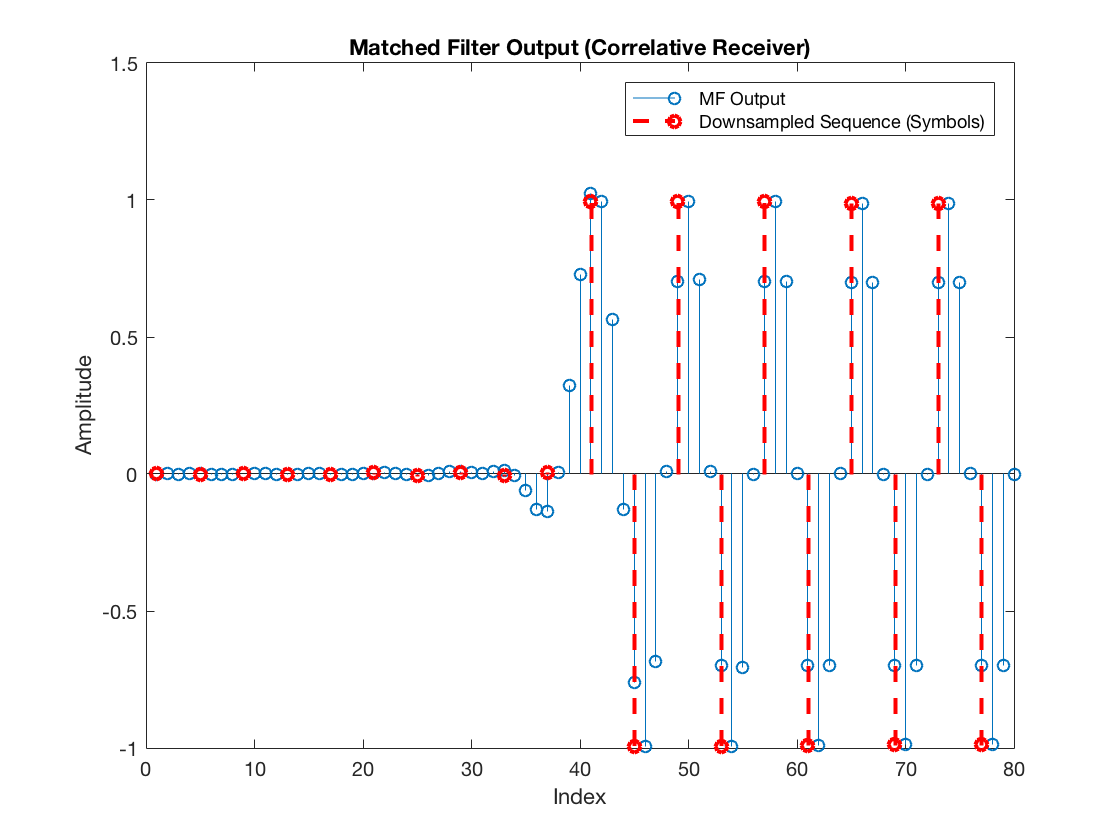So the symbols passed to the PAM decision module are:

figure
stem(rxSym)
title('Symbol Sequence')
xlabel('Symbol Index')
ylabel('Amplitude')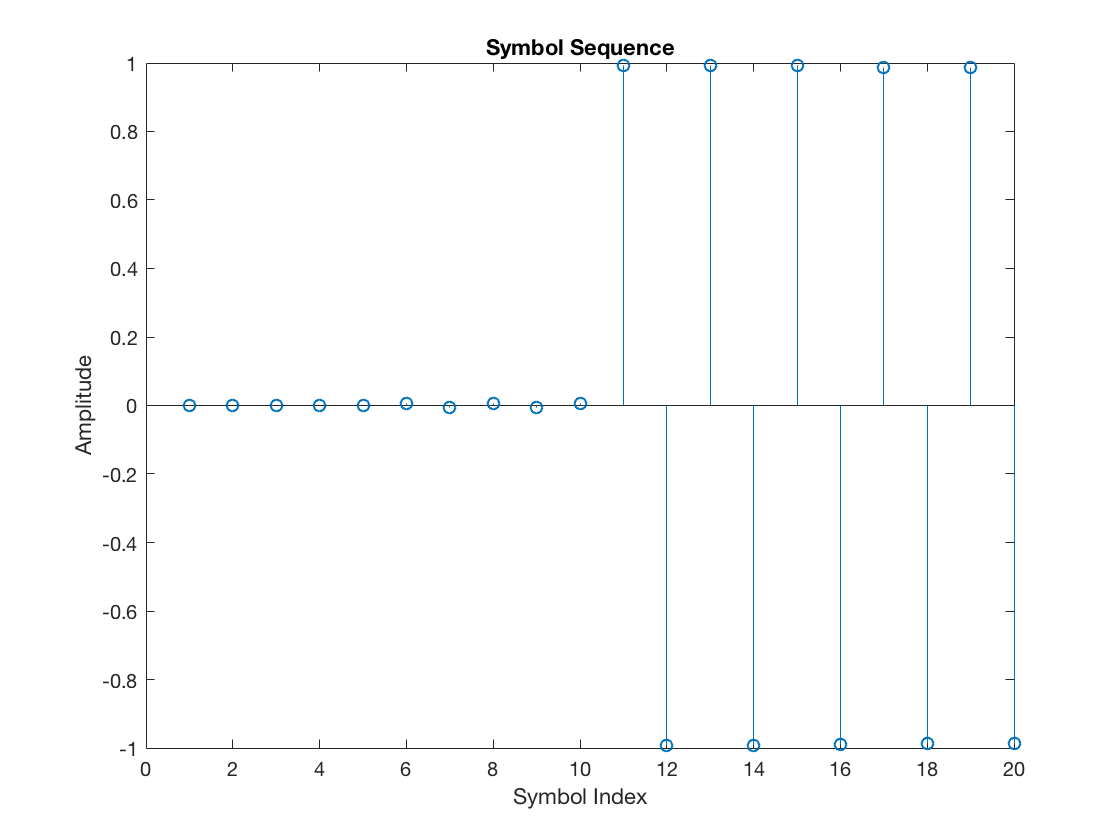And, again, skiping the raised cosine pulse delay, the corresponding scatter plot becomes:

figure
plot(complex(rxSym(rcDelay+1:end)), 'o')
grid on
xlim([-1.5 1.5])
title('Rx Scatterplot')
xlabel('In-phase (I)')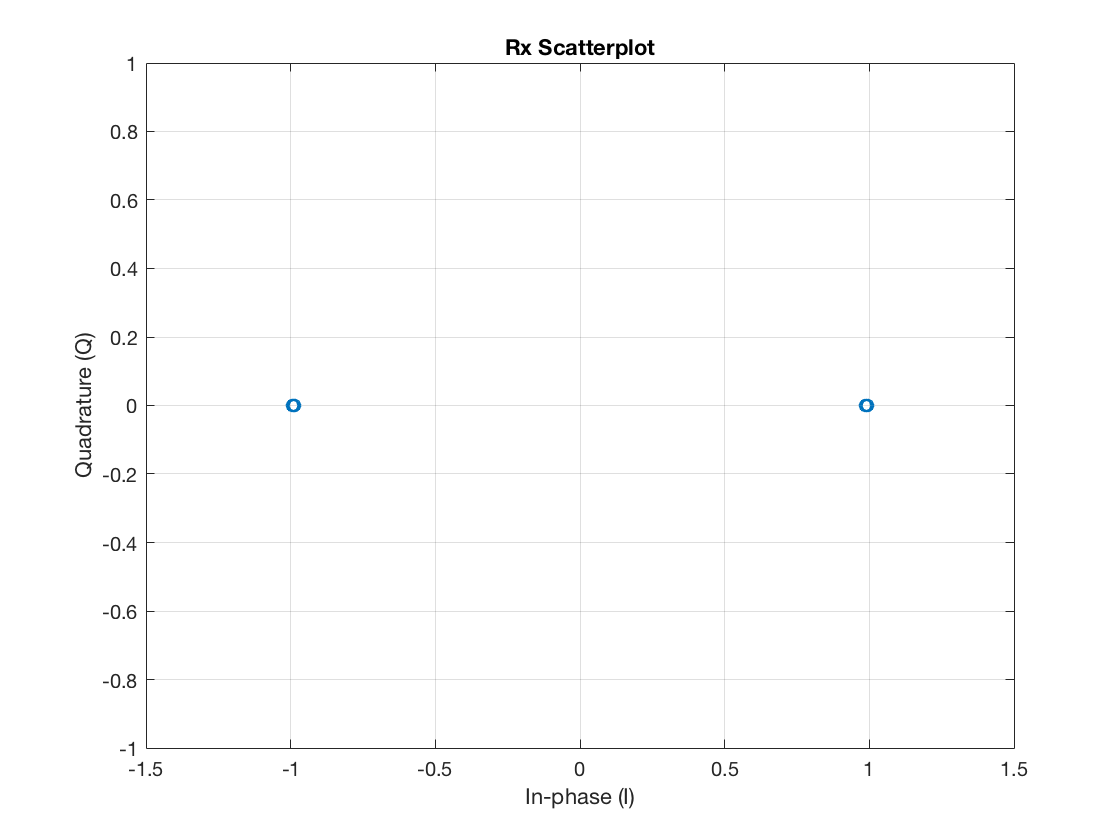Perfect, isn’t it? So, all the receiver needs is to be able to somehow find the exact instants where the zero-crossings of the pulse shaping function (convolved with each individual symbol) are located. This is done by the timing error detector (TED) in a timing recovery loop. Let’s see two possible approaches next.

Derivative Matched Filter (dMF)

One of the possible TED schemes is the so-called maximum likelihood TED (ML-TED). It employs a derivative matched filter, which, as the name implies, is a filter whose output corresponds to the derivative of the matched filter. And why would we be interested in the derivative? It is because the derivative approaches zero at the peaks (because the slope transitions from positive to negative in this point). Let’s see how this looks like in practice.

First we design the dMF. There is an important observation prior to doing so. There are several ways of approximating a derivative in discrete time. The main difference lies in the resulting frequency response of the filter and, more specifically, how it affects noise. One approach that avoids enhancing high-frequency noise is the central differences differentiator (refer to this informative post). Therefore, we choose this approach in what follows:

dMF design

h = [0.5 0 -0.5]; % central-differences kernel function
central_diff_mf = conv(h, hrx);

% Skip the kernel delay
dmf = central_diff_mf(2:1+length(hrx));

figure
plot(hrx)
hold on, grid on
plot(dmf, 'r')
legend('MF', 'dMF')
title('MF vs. dMF')
xlabel('Index')
ylabel('Amplitude')
hold off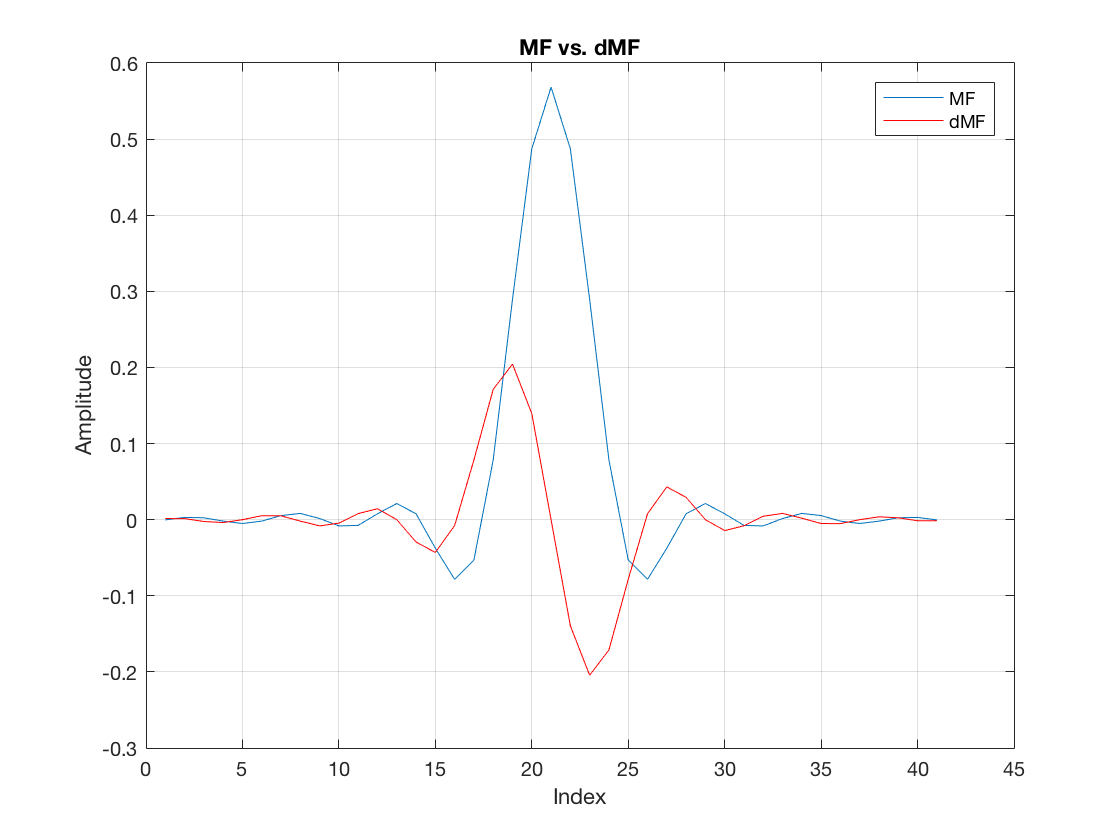So, we can see that the very center of the MF (the peak) is aligned with a zero amplitude at the dMF.

Maximum likelihood Timing Error Detector (ML-TED)

In the ML-TED scheme, the dMF concurrently filters the same sequence that the MF filters at the receiver. Therefore, this is how the dMF output looks like:

dmfOutput = filter(dmf, 1, rxDelayed);

figure
stem(dmfOutput)
title('Derivative Matched Filter Output')
xlabel('Index')
ylabel('Amplitude')

rxSym = downsample(mfOutput, L, timeOffset);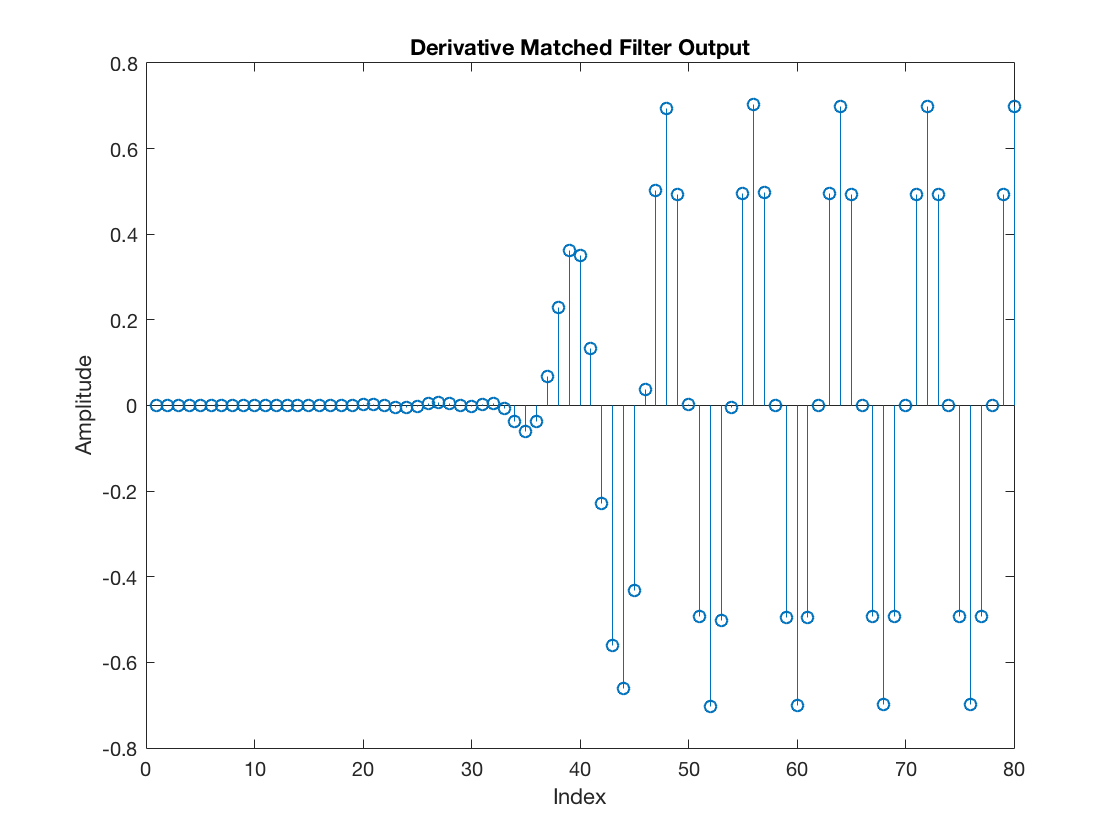Furthermore, the ML-TED module at the symbol timing recovery loop extracts a timing error exactly at the same instant when a sample from the MF output is retained as a symbol. The result is shown next. In particular, we can contrast the timing errors that would result from the dMF for the two receiver timing cases shown earlier, the one without knowledge of the channel-introduced timing offset and the one that does know the exact timing instants.

dmfDownsampled_nosync = downsample(dmfOutput, L);
dmfDownsampled_withsync = downsample(dmfOutput, L, timeOffset);

% And just for illustration purposes
figure
stem(dmfDownsampled_nosync)
hold on
stem(dmfDownsampled_withsync, '--r', 'LineWidth', 2)
xlabel('Symbol Index')
ylabel('Amplitude')
legend('No Symbol Timing Sync', 'With Sync')
title('Downsampled dMF output')
hold off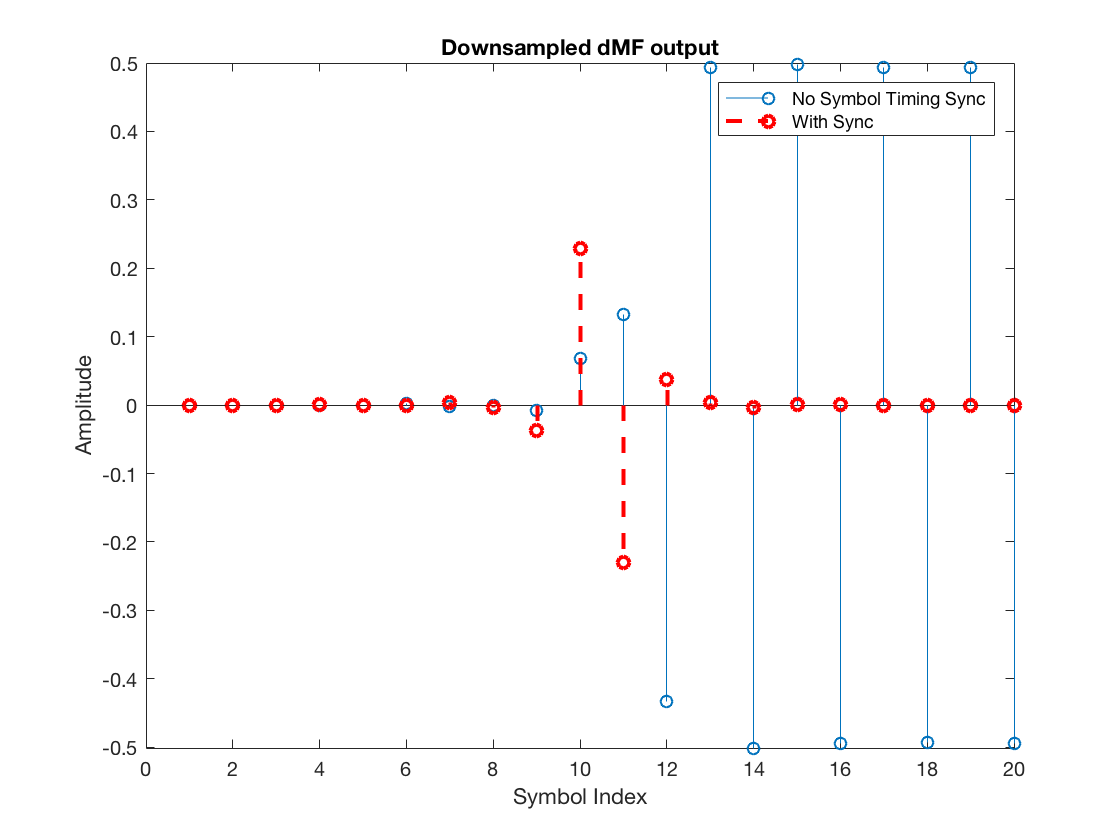Note that the timing error has significant amplitude for the receiver that has not yet acquired the knowledge of the symbol timing offset, whereas it is practically null for the receiver that already perfectly knows the symbol timing offset. Hence, we can conclude that this dMF can be very useful to us, given our goal of discovering the misalignment with respect to the proper symbol timing instants (aligned with the RC zero-crossings).

Nonetheless, there is a very important observation for this particular ML-TED scheme. It has only worked above because we forced the transmit symbols to be alternating +1, -1, +1, -1, and so on. Remember that I told this would be important to keep in mind? Here it is. If that was not the case, that is, for a practical random symbol stream, the ML-TED output would be very noisy. This noise has a specific name in the context of symbol timing recovery, it is called self-noise . Other TED schemes, such as the zero-crossing TED (ZC-TED) do not suffer from this noise.

Before advancing into the ZC-TED, there is one final remark. The ML-TED timing error outputs above are not complete. It does not suffice to extract the raw downsampled values of the dMF. A sign correction must be applied to these values. A very good explanation is provided with respect to Fig. 8.2.2 in , but let’s summarize the idea here.

Essentially, the receiver that does not apply any timing offset correction is equivalently guessing that the timing offset is$\hat{\tau} = 0$. However, there is a timing offset$\tau = 1$, so the timing error is$\tau_e = \tau - \hat{\tau} = 1$. The solution is to increase the guess$\hat{\tau}$, until it yields a zero error ($\tau_e = 0$).

When the MF output is transitioning from a -1 symbol to a +1 symbol, the slope of the output is positive. Therefore, if we extract a sample from the dMF output using$\hat{\tau} = 0$, then we get a positive value. This value tells the receiver’s timing recovery loop that the current guess$\hat{\tau}$ must be increased (because the error is positive). In the contrary case, when the MF output is transitioning from a +1 to -1, the slope of the MF output is negative, so the extracted dMF sample is negative. This indicates that the current guess$\hat{\tau}$ must be decreased.

Nonetheless, since there is a timing offset$\tau = 1$ and the current guess is$\hat{\tau} = 0$, the correct information in this case would be to increase the guess$\hat{\tau}$. Note, then, that if we just multiply the dMF output by the detected symbol, it would be multiplied by +1 in the positive transition (-1 to +1, because -1 is the previous symbol and +1 is the current symbol), and multiplied by -1 in the negative transition (from +1 to -1). Therefore, it would always yield a positive value, according to what we expect.

Of course, because$\tau$ is between 0 and L-1, the guess$\hat{\tau}$ must be modulo-L. So, for example, if it is currently$\hat{\tau} = 0$, and$L = 4$, an unitary decrease would lead to$\hat{\tau} = 3$.

Once sign correction (using the detected symbols) is applied, the following result is obtained:

% Detected Symbols

% First do PAM-demodulation
rxdata_nosync   = pamdemod(downsample(mfOutput, L), M);
rxdata_withsync = pamdemod(downsample(mfOutput, L, timeOffset), M);
% Then, regenerate the corresponding constellation symbols
decSym_nosync   = real(pammod(rxdata_nosync, M));
decSym_withsync = real(pammod(rxdata_withsync, M));

% TED Output
e_nosync       = decSym_nosync .* dmfDownsampled_nosync;
e_withsync     = decSym_withsync .* dmfDownsampled_withsync;

% And just for illustration purposes
figure
stem(e_nosync)
hold on
stem(e_withsync, '--r', 'LineWidth', 2)
xlabel('Symbol Index')
ylabel('Amplitude')
legend('No Symbol Timing Sync', 'With Sync')
title('ML-TED Output - Timing Errors')
hold off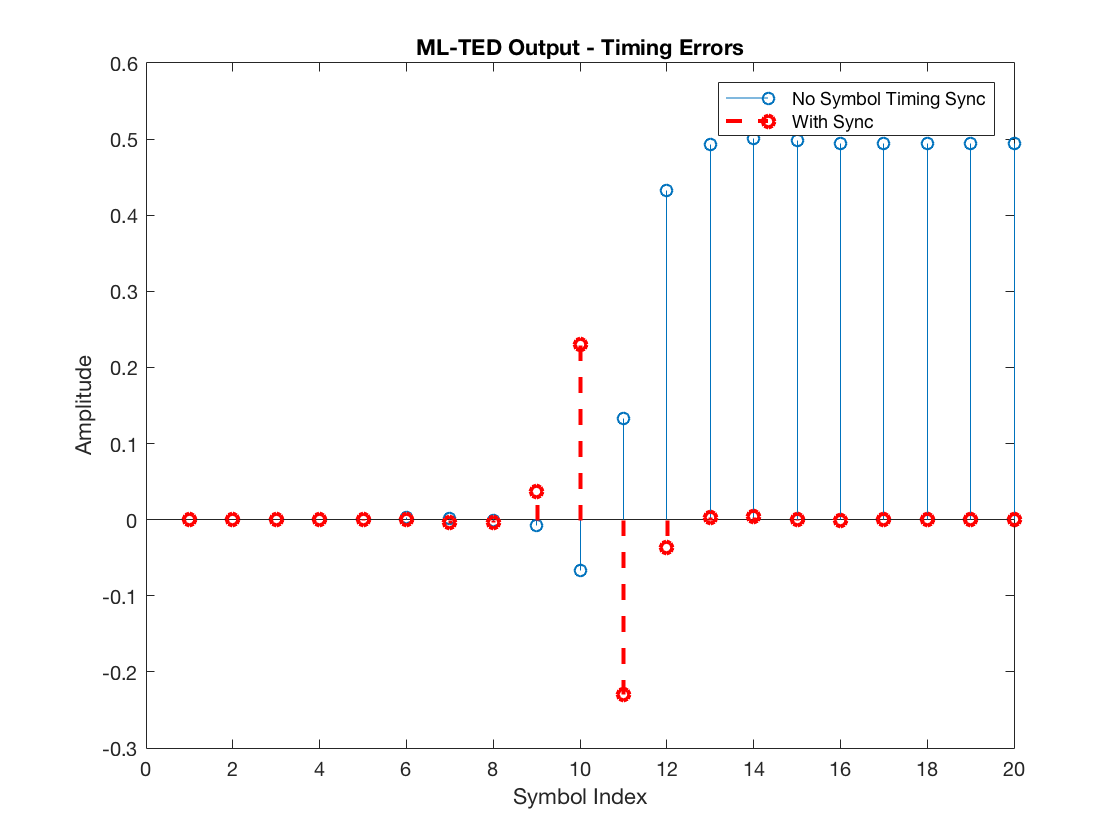Note the TED output for the system that has not yet acquired the correct symbol timing offset is positive, in compliance to the fact that the current guess$\hat{\tau} = 0$ must be increased to approach the actual$\tau = 1$.

Zero-crossing Timing Error Detector (ZC-TED)

To conclude this tutorial, let’s investigate the ZC-TED, which, as stated earlier, does a better job because it avoids self-noise. This TED scheme doesn’t need a dMF filter. Instead, it operates solely by using the samples of the regular MF output.

The principle exploited by the ZC-TED is that when the MF output transitions from +1 to -1 or vice versa, it crosses the zero amplitude. In particular, because there are L-1 samples between each two consecutive symbols, it is expected that the zero-crossing is near (if not exactly in) the middle index between these L-1 samples. Therefore, the receiver can constantly observe this sample located in the midpoint between a transition and use this value as the timing error. If it is effectively aligned with the zero-crossing, then the error is zero and the symbol timing offset has been acquired. If it is not zero, then the current guess$\hat{\tau}$ of the symbol timing offset must be adjusted.

To understand the adjustment applied to the current guess$\hat{\tau}$, we consider the two possible scenarios, a positive transition (from -1 to +1) and a negative transition (from +1 to -1). First, let’s observe a negative transition and closer inspect the samples that are between the two symbols (+1 and -1) in the system that has not yet acquired the correct timing offset, namely whose current timing offset guess is$\hat{\tau} = 0$.

figure
stem(mfOutput(41:41+L))
hold on
plot(mfOutput(41:41+L))
hold off
xlabel('Index')
ylabel('Amplitude')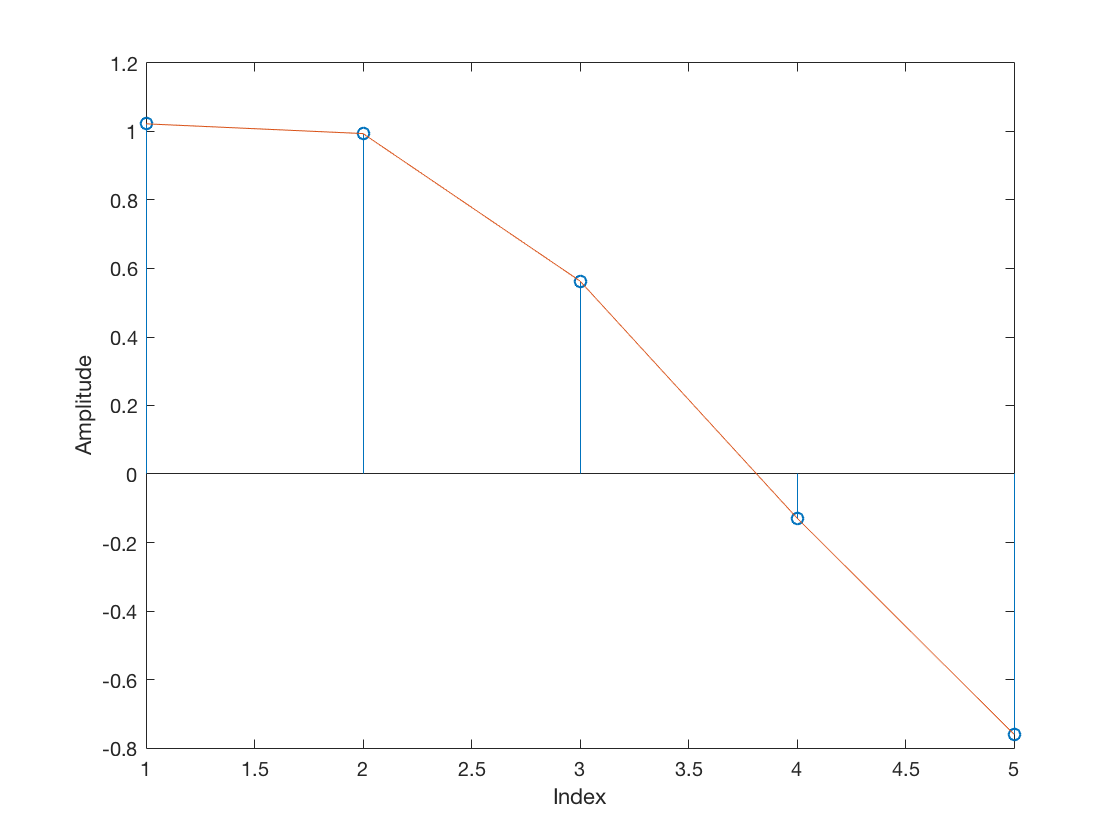Note the midpoint sample (at index 3) is positive. In the contrary case, that is, for a positive transition (from -1 to +1):

figure
stem(mfOutput(45:45+L))
hold on
plot(mfOutput(45:45+L))
hold off
xlabel('Index')
ylabel('Amplitude')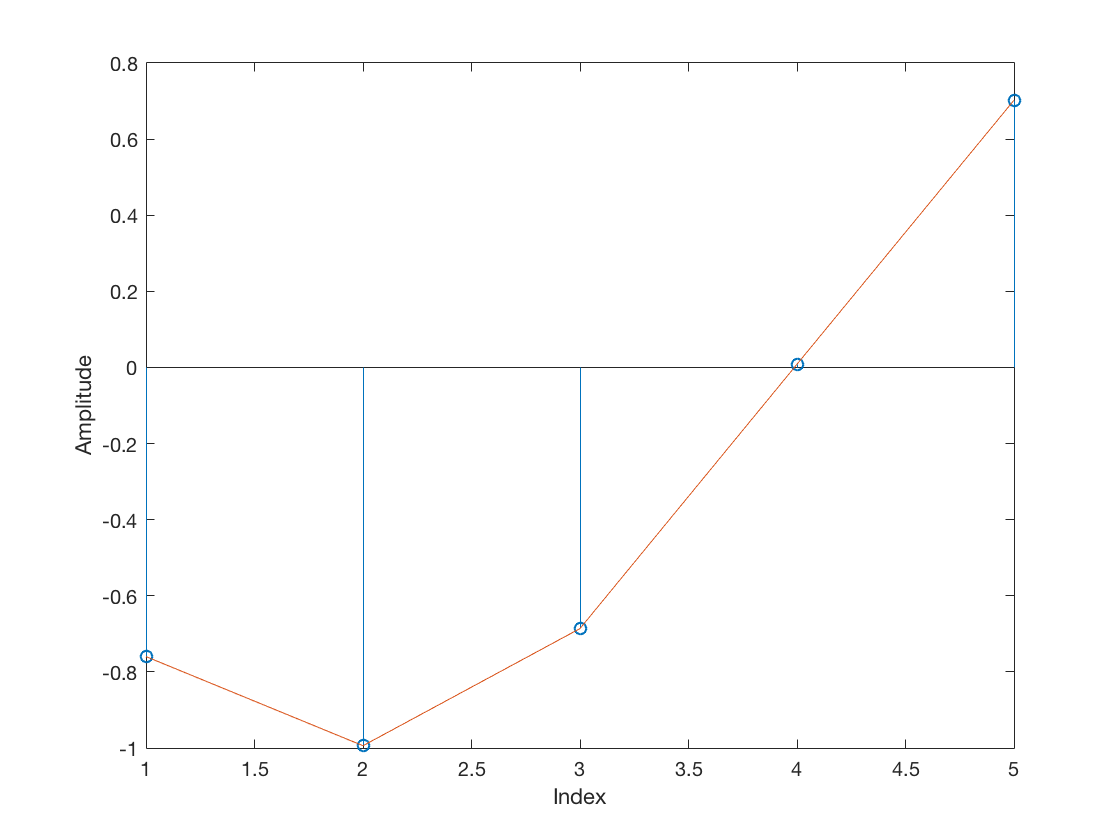The midpoint sample is negative. Hence, the proper way of sign-correcting this sample is by multiplying it by the difference$\hat{a}(k-1) - \hat{a(k)}$ between the previous symbol and the current symbol. This difference can be based on the actual symbols, when known at the receiver side (data-aided synchronization), or based on the decisions (decision based synchronization).

Next, we can observe the ZC-TED output for the two systems.

% Midpoint samples
midSamples_nosync     = mfOutput((L/2 + 1):L:end);
midSamples_withsync   = mfOutput((L/2 + 1 + timeOffset):L:end);

% Sign correction values based on symbol decisions:
signcorrection_nosync   = [-diff(decSym_nosync), 0];
signcorrection_withsync = [-diff(decSym_withsync), 0];
% Note: the padded zero is just for the signcorrection vector
% to have a length equivalent to the the vector of midsamples.

% ZC-TED output:
zcted_nosync   = midSamples_nosync .* signcorrection_nosync;
zcted_withsync = midSamples_withsync .* signcorrection_withsync;

% Plot
figure
stem(zcted_nosync)
hold on
stem(zcted_withsync, '--r', 'LineWidth', 2)
hold off
xlabel('Symbol Index')
ylabel('Amplitude')
legend('No Symbol Timing Sync', 'With Sync')
title('ZC-TED Output - Timing Errors')So, it is possible to see that the ZC-TED yields proper correction values. A positive value for the receiver that has not yet acquired symbol timing sync, and a zero value for the receiver that already locked to the corrected symbol timing offset.

Finally, observe the reason why the ZC-TED scheme does not suffer from self-noise. It is because the sign-correction term$\hat{a}(k-1) - \hat{a(k)}$ is$0$ when two consecutive symbols are equal, in this case, +1 and +1, or -1 and -1. Therefore, the scheme simply does not mandate any timing correction when there is no zero-crossing in between the two symbols of interest. In contrast, with the ML-TED, even though it requires a data transition to yield a reasonable timing error estimate, it does not eliminate the timing error estimate when the consecutive symbols are equal, leading to self-noise.

Final Remarks

This was an introductory tutorial to the concept of symbol timing synchronization. The simulation provided in http://github.com/igorauad/symbol_timing_sync implements a complete timing recovery loop, featured with a PI controller, two alternative interpolators (a linear interpolator and a polyphase interpolator) and the clever modulo-1 counter scheme of  that serves as the interpolator controller. Furthermore, it has one feature that can be really useful while learning how those loops behave: it has debug options for launching a time scope and showing “in real-time” (over each iteration of the simulation) the effects of the timing recovery corrections in the constellation. Feel free to experiment with it and even contribute to the repository.

References

 Rice, Michael. Digital Communications: A Discrete-Time Approach. Upper Saddle River, NJ: Prentice Hall, 2009.